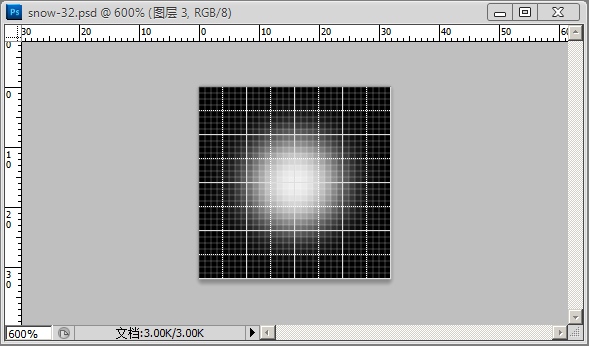# three.js入门及飘雪实现

three.js是一个JavaScript的3D库，可用于构建WebGL应用。2011年写过一篇《一个二进制的Web新世界》，从那时起就一直断断续续有接触这方面的资料。本文是three.js的一些入门，并用其实现一个飘雪的效果

``````var scene = new THREE.Scene();
var camera = new THREE.PerspectiveCamera(75, window.innerWidth / window.innerHeight, 1, 2000);
renderer = new THREE.WebGLRenderer();
renderer.render(scene, camera);
````````````var container, camera, scene, renderer, particles = [], material, map, textureLoader;
var halfX = window.innerWidth / 2;
var halfY = window.innerHeight / 2;
var mouseX = 0,
mouseY = 0;
var fallSpeen = 2;
var amount = 1000;

function randomRange(min, max) {
return ((Math.random() * (max - min)) + min);
}
``````

``````container = document.createElement('div');
document.body.appendChild(container);
camera = new THREE.PerspectiveCamera(75, window.innerWidth / window.innerHeight, 1, 2000);
camera.position.z = 100;
scene = new THREE.Scene();
``````

``````textureLoader = new THREE.TextureLoader();
material = new THREE.SpriteMaterial({map: map});
``````

``````for ( i = 0; i < amount; i ++ ){
var particle = new THREE.Sprite( material );
var randomScale = randomRange(10,20)

particle.position.x = randomRange(-1000, 1000);
particle.position.y = randomRange(-1000, 1000);
particle.position.z = randomRange(-1000, 1000);
particle.scale.x = particle.scale.y = particle.scale.z = randomScale;
particle.v = new THREE.Vector3(0, -fallSpeen, 0);
particle.v.z = (1 * randomRange(-1, 1));
particle.v.x = (1 * randomRange(-1, 1));

particles.push(particle);
}
``````

``````renderer = new THREE.WebGLRenderer({alpha: true});
renderer.setPixelRatio(window.devicePixelRatio);
renderer.setSize(window.innerWidth, window.innerHeight);
container.appendChild(renderer.domElement);

animate();
``````

`animate()`中，通过`requestAnimationFrame()`方法持续调用`render()`来反复渲染形成动画。

``````function animate() {
requestAnimationFrame(animate);
render();
}
``````

``````function render() {
for(var i = 0; i < particles.length; i++){
var particle = particles[i];
var pp = particle.position;

if(pp.y < -1000) pp.y = 1000;
if(pp.x > 1000) pp.x = -1000;
else if(pp.x < -1000) pp.x = 1000;
if(pp.z > 1000) pp.z=-1000;
else if(pp.z < -1000) pp.z = 1000;
}
renderer.render( scene, camera );
}
``````

three.js的大部分动画就有类似这样的流程，初始化场景，相机，添加物体，指定光照和材质，然后渲染。如果需要动画则重复渲染更新，通过`requestAnimationFrame()`方法浏览器已经可以获得比`setInterval()`好的多的流畅度，通过WebGL渲染器替代Canvas渲染器，可以使用显卡而非CPU来渲染场景，获得更低的资源占用。而这些就是three.js的最基本的内容。

Disqus提供评论支持，如果评论长时间未加载，请飞跃长城。